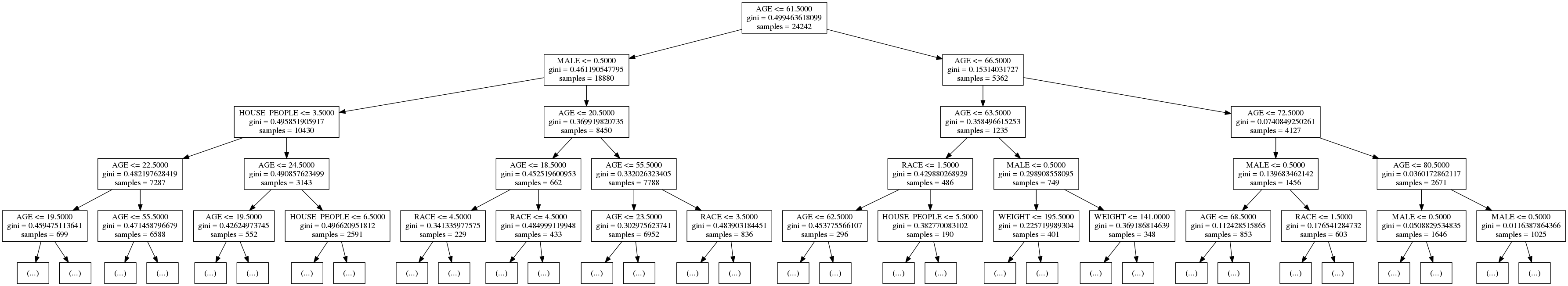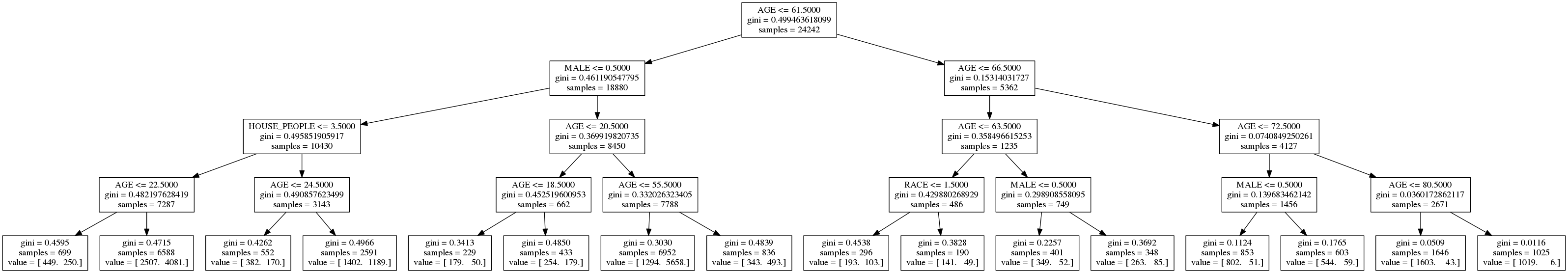# samucoder

Samuel M.H. 's technological blog

Notebook

# Running a Classification Tree

Author: Samuel M.H. <samuel.mh@gmail.com> Date: 27-03-2016

## Instructions

This week’s assignment involves decision trees, and more specifically, classification trees. Decision trees are predictive models that allow for a data driven exploration of nonlinear relationships and interactions among many explanatory variables in predicting a response or target variable. When the response variable is categorical (two levels), the model is a called a classification tree. Explanatory variables can be either quantitative, categorical or both. Decision trees create segmentations or subgroups in the data, by applying a series of simple rules or criteria over and over again which choose variable constellations that best predict the response (i.e. target) variable.

### Run a Classification Tree.

You will need to perform a decision tree analysis to test nonlinear relationships among a series of explanatory variables and a binary, categorical response variable.

## What to Submit

Following completion of the steps described above, create a blog entry where you submit syntax used to run a Classification Tree (copied and pasted from your program) along with corresponding output and a few sentences of interpretation. Please note that your reviewers should NOT be required to download any files in order to complete the review.

## Intro

This week I will look for nonlinear relationships in order to predict if a person works 35 or more hours a week. This will be done with decision trees.

## Dataset

### Variables

• Response:

• WORK35 -> S1Q7A1: present situation includes working full time (35+ hours a week). Categorical yes/no.
• Explanatory:

• AGE -> AGE: age (years).
• S1Q24LB -> WEIGHT: weight (pounds).
• NUMPERS -> HOUSE_PEOPLE: number of persons in household.
• ETHRACE2A -> RACE: imputed race/ethnicity (5 groups, reference group=1,white).
• SEX -> MALE: gender (2 groups).
• S10Q1A63 -> CHANGE_MIND: change mind about things depending on people you're with or what read or saw on tv (2 groups).
In :
%pylab inline

import numpy as np
import pandas as pd

from sklearn.cross_validation import train_test_split
from sklearn.tree import DecisionTreeClassifier
from sklearn.metrics import classification_report
import sklearn.metrics

#Visualization
from sklearn import tree #Dot format export
from IPython.display import Image #See into notebook
from io import BytesIO #Temporal file, don't use StringIO due to unicode
import pydot #Dot interface

pylab.rcParams['figure.figsize'] = (15, 8)

Populating the interactive namespace from numpy and matplotlib



## Data

In :
# Load data

In :
# Custom dataframe
df = pd.DataFrame()

# Response variable
df['WORK35'] = data['S1Q7A1'].replace(' ',np.NaN).replace('2','0').astype(float)

# Explanatory variables
df['AGE'] = data['AGE'].replace(' ',np.NaN).replace('98',np.NaN).astype(float)
df['WEIGHT'] = data['S1Q24LB'].replace(' ',np.NaN).replace('999',np.NaN).astype(float)
df['HOUSE_PEOPLE'] = data['NUMPERS'].replace(' ',np.NaN).astype(float)
df['RACE'] = data['ETHRACE2A'].replace(' ',np.NaN).astype('category')
df['MALE'] = data['SEX'].replace(' ',np.NaN).replace('2','0').astype('category')
df['CHANGE_MIND'] = data['S10Q1A63'].replace(' ',np.NaN).replace('9',np.NaN).replace('2','0').astype('
category')

df = df.dropna()

/usr/lib/python2.7/dist-packages/pandas/core/internals.py:4417: FutureWarning: elementwise comparison failed; returning scalar instead, but in the future will perform elementwise comparison
comp = (nn == nn_at)



### Data summaries: counts and descriptions

In :
df['WORK35'].value_counts()

Out:
1    20942
0    19462
Name: WORK35, dtype: int64

In :
pd.crosstab(df['WORK35'],df['RACE'])

Out:
RACE 1 2 3 4 5
WORK35
0 11406 3629 333 557 3537
1 11617 3999 321 681 4324
In :
pd.crosstab(df['WORK35'],df['MALE'])

Out:
MALE 1 0
WORK35
0 6509 12953
1 11035 9907
In :
pd.crosstab(df['WORK35'],df['CHANGE_MIND'])

Out:
CHANGE_MIND 1.0 0
WORK35
0 2887 16575
1 2867 18075
In :
df[['AGE','WEIGHT','HOUSE_PEOPLE']].describe().round(3)

Out:
AGE WEIGHT HOUSE_PEOPLE
count 40404.000 40404.000 40404.000
mean 46.277 170.754 2.521
std 18.157 41.376 1.495
min 18.000 62.000 1.000
25% 32.000 140.000 1.000
50% 44.000 165.000 2.000
75% 59.000 193.000 3.000
max 97.000 500.000 17.000

### Split: train, test

In :
TARGET = 'WORK35'
PREDICTORS = list(df.columns)
PREDICTORS.remove(TARGET)

df_target = df[TARGET]
df_predictors = df[PREDICTORS]

train_target, test_target, train_predictors, test_predictors = train_test_split(df_target, df_predictors, test_size=0.4, random_state=42)

print('Samples train: {0}'.format(len(train_target)))
print('Samples test:  {0}'.format(len(test_target)))

Samples train: 24242
Samples test:  16162



## Model

In :
model1 = DecisionTreeClassifier()
model1 = model1.fit(train_predictors, train_target)


### Metrics

In :
predictions=model1.predict(test_predictors)

print('Confusion matrix [[TP,FP],[FN, TN]]')
print(sklearn.metrics.confusion_matrix(test_target,predictions))

print('\nAccuracy')
print(sklearn.metrics.accuracy_score(test_target, predictions))

Confusion matrix [[TP,FP],[FN, TN]]
[[5168 2570]
[3036 5388]]

Accuracy
0.653136987997



$$ACC = \frac{TP+TN}{P+N}$$

### Visualization

In :
print('Tree depth: {0} nodes'.format(model1.tree_.max_depth))

Tree depth: 35 nodes


In :
out = BytesIO()
tree.export_graphviz(model1, out_file=out, max_depth=4, feature_names=PREDICTORS)
graph=pydot.graph_from_dot_data(out.getvalue())
Image(graph.create_png())

Out:### Thoughts

The accuracy of the model is only 0.655, which is slightly better than a random choose of 0.5. I see there is a problem, and maybe the depth of the tree, 35 nodes, is a symbol of overfitting and is its generalization power. Lets try a model limiting the maximum depth to only 4 nodes.

## Improving the model

In :
model2 = DecisionTreeClassifier(max_depth=4)
model2 = model2.fit(train_predictors, train_target)


### Metrics

In :
predictions = model2.predict(test_predictors)

print('Confusion matrix [[TP,FP],[FN, TN]]')
print(sklearn.metrics.confusion_matrix(test_target,predictions))

print('\nAccuracy')
print(sklearn.metrics.accuracy_score(test_target, predictions))

Confusion matrix [[TP,FP],[FN, TN]]
[[4954 2784]
[1545 6879]]

Accuracy
0.73214948645



### Visualization

In :
out = BytesIO()
tree.export_graphviz(model2, out_file=out, feature_names=PREDICTORS)
graph = pydot.graph_from_dot_data(out.getvalue())
Image(graph.create_png())

Out:### Conclusion

With this improved model, the accuracy increases to 0.732 which is better than the overfitted initial model.

It is possible to see the age is the most important factor to determine if a person works more than 35 hours a week. When a person is older than 72.5 years it is more likely they work less than 35 hours a week. And this likelihood decreases until the person is 66.5 years old.

Between 63.5 and 66.5 years, the gender seems to be determinant as there are more males working.

There is a curious thing with people between 61.5 and 63.5, that is it seems whites are more likely to have a full time job.

Other interesting relationship found is for males between 20.5 and 55.5. The people with a full time job are 4 times more than the people without.

It is important to know the meaning of the giny impurity criterion and take into consideration the number of samples when interpreting my statements as the closer the gini is to 0.5 the weaker the statement is supported. And the the same happens with the number of samples when they appoach to 0. The more data, the more confident I can be.

For females under 61.5 years I cannot say much as all the gini indices are close to 0.5.

Summing up, this is a bad model but useful example to explore data with a classification tree. In order to improve the model, I'll have to add more features/predictors. Other thing I haven't been able to do is successfully use a multilevel categorical predictor, i.e. the race. That means with an equal criterion in the split instead of a bigger-lesser one. To achieve this behaviour I should split the feature into n-binary features meaning each one if the person belongs to that race.